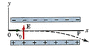# Electric Field Projectile Motion

physicsss
At what angle will the electrons leave the uniform electric field at the end of the parallel plates? Assume the plates are 5.4 cm long and E = 5.0 x 10^3 N/C. Ignore fringing of the field. (counterclockwise from the x-axis is positive)

I know the horizontal position is given by x=V0*t and
y= -((e*E)/(2*m*V0^2))(x^2) where e is charge of the electron and x is the horizontal position...

#### Attachments

•21-40.gif
4.2 KB · Views: 551